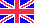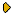Журнал Экспериментальной и Теоретической Физики
 НАЧАЛО | ПОИСК | ДЛЯ АВТОРОВ | ПОМОЩЬОбщая информация о журналеЗолотые страницыАдреса редакцииСодержание журналаСообщения редакцииПравила для авторовЗагрузить статьюПроверить статус статьиЖЭТФ, Том 118, Вып. 3, стр. 730 (Сентябрь 2000) (Английский перевод - JETP, Vol. 91, No 3, September 2000 доступен on-line на www.springer.com ) LINEAR RELAXATION PROCESSES GOVERNED BY FRACTIONAL SYMMETRIC KINETIC EQUATIONS Chechkin A.V., Gonchar V.Yu. Поступила в редакцию: 4 Ноября 1999 PACS: 05.10.Gg, 05.40.Fb We derive the fractional symmetric Fokker-Planck and Einstein-Smoluchowski kinetic equations that describe the evolution of systems influenced by stochastic forces distributed with stable probability laws. These equations generalize the known kinetic equations of the Brownian motion theory and involve symmetric fractional derivatives with respect to velocity and space variables. With the help of these equations, we study analytically the linear relaxation processes in the force-free case and for the linear oscillator. For a weakly damped oscillator, we also obtain a kinetic equation for the distribution in slow variables. Linear relaxation processes are also studied numerically by solving the corresponding Langevin equations with the source given by a discrete-time approximation to the white Levy noise. Numerical and analytical results agree quantitatively.
Сообщить о технических проблемах